# How to perform Granger causality test in STATA?

A previous article (Lag selection and cointegration test in VAR with two variables) in this module demonstrated the application of cointegration test in time series analysis. Applying Granger causality test in addition to cointegration test like Vector Autoregression (VAR) helps detect the direction of causality. It also helps to identify which variable acts as a determining factor for another variable. This article shows how to apply Granger causality test in STATA.

VAR model includes past values of other series to the series’ own history. Granger causality in a VAR model implies a correlation between current values of one variable and the past values of other variables. For example, consider two variables X and Y.  If lagged values of X and Y can predict X, then Y will Granger-cause X. Conversely, if X influences Y, then X Granger-causes Y. This is called unidirectional causality because of one variable Granger-causes another variable. In some cases, both variables X and Y are found to be influenced by the other’s lagged values in addition to its own. This leads to a bidirectional causality.

## Declare time variable using VAR in STATA

Before performing the Granger causality test, declare the ‘time’ variable as follows. This article takes into consideration the same three variables as previous articles; Gross Domestic Product (GDP), Private Final Consumption (PFC) and GFC (Gross Fixed Capital Formation) of India. The time period is 1997 to 2018.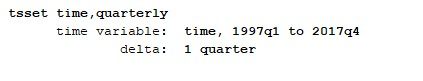Figure 1: Setting of time series in VAR model in STATA

To perform VAR in STATA, follow these steps:

• Go to ‘Statistics’ on Result window
• Go to ‘Multivariate time series’
• Click on ‘Vector autoregression (VAR)’.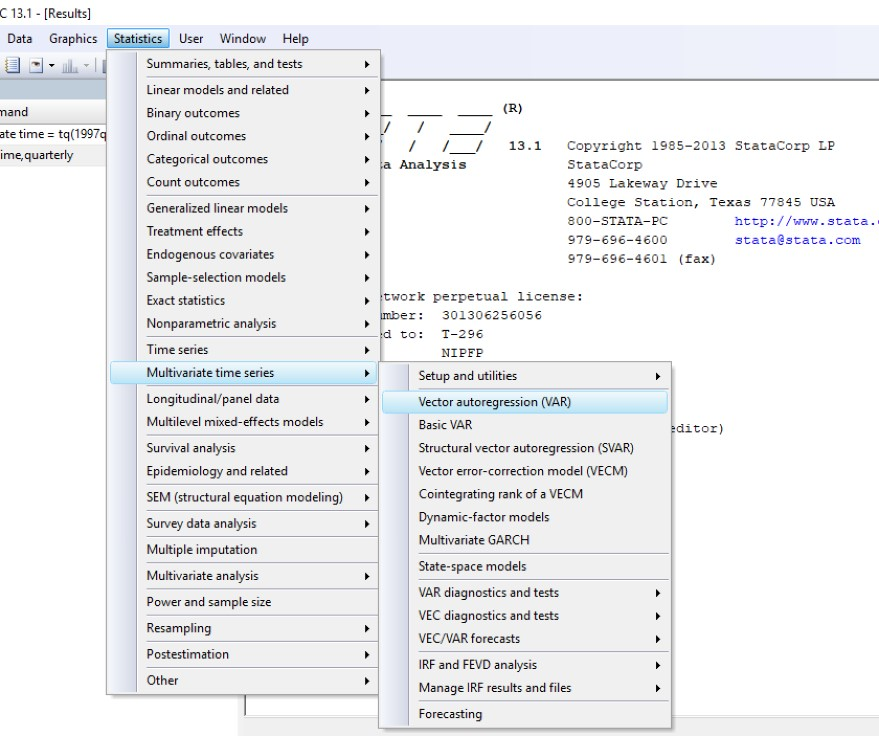Figure 2: Performing VAR in STATA

The below figure will appear. Choose the dependent variables in the box, i.e. gdp, gfcf, pfce. Select number of lags, i.e. 2. Then click on ‘OK’.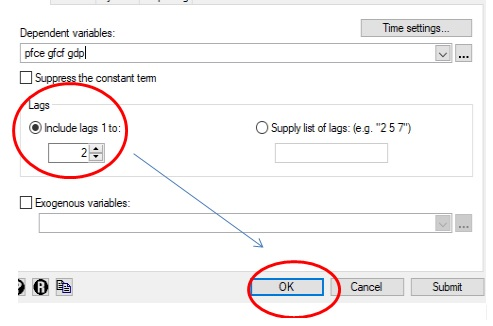Figure 3: Lag selection for VAR in STATA

Alternatively, use the following STATA command for VAR:

`VAR pfcegfcfgdp, lags(1/2)`

The below results will appear.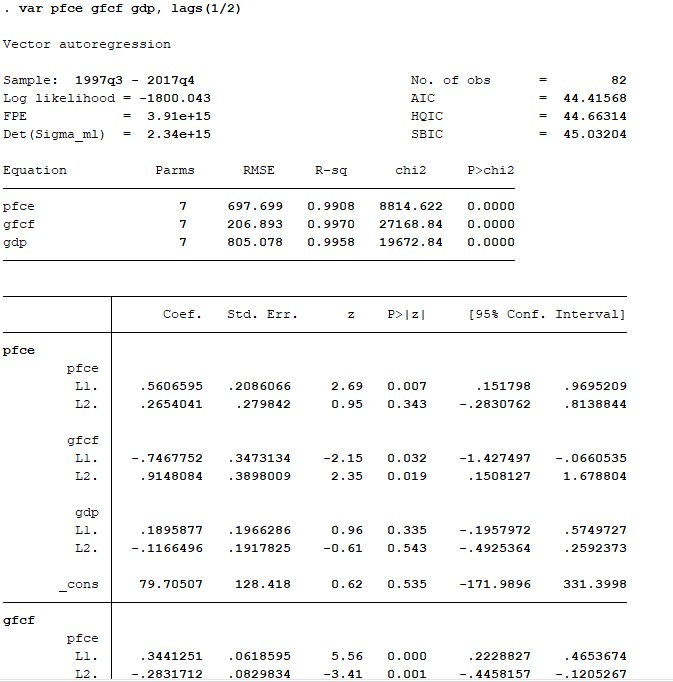Figure 4: Results of VAR in STATA

Next, perform the Granger causality test to examine the direction of causality between the variables.

## Granger causality test

For executing the Granger causality test in STATA, follow these steps:

1. Go to ‘Statistics’.
2. Click on ’Multivariate time series’.
3. Select ‘VAR diagnostics and tests’.
4. Choose ‘Granger causality tests’.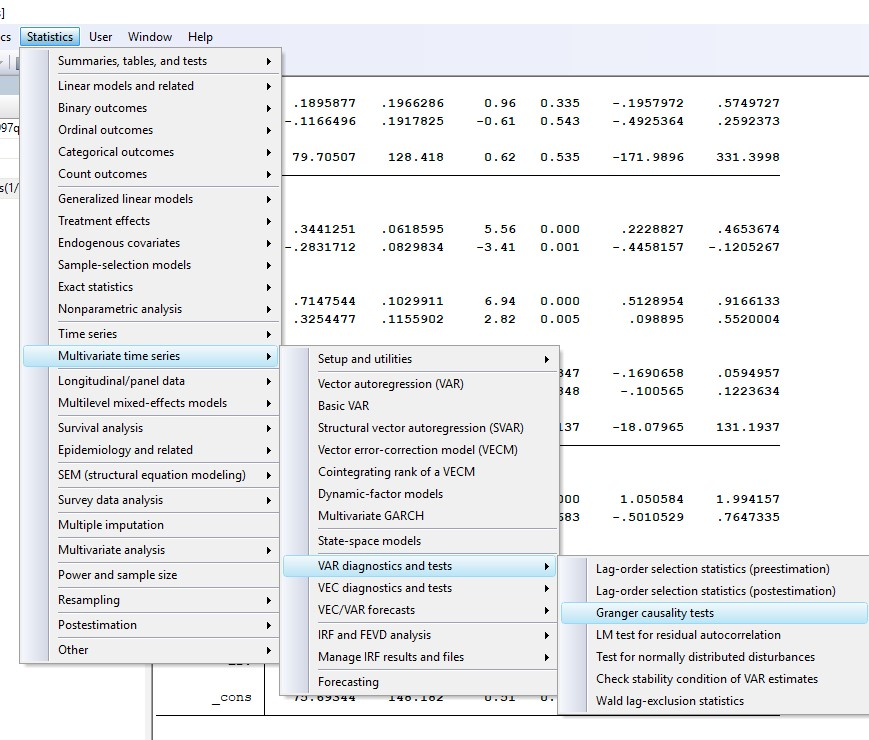Figure 5: Performing the Granger causality test in STATA

The below figure will appear. Select ‘Use active or svar results’ and click on ‘OK’.Figure 6: Granger causality test in STATA

The null hypothesis for Granger causality test is:

• First equation: Lagged values of gfcf and gdp do not cause pfce.
• Second equation: Lagged values of pfce and gdp do not cause gfcf.
• Third equation: Lagged values of pfce and gfcf do not cause gdp.

## Results

The results of Granger causality test will appear.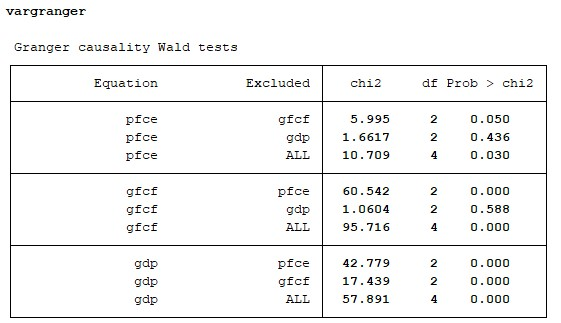Figure 7: Results of Granger causality test in STATA

### First row

The first row of the above figure shows that lagged values of gfcf cause pfce as p-value is equal to 0.05. However, because of the p value (0.436 > 0.05), lagged values of gdp do not cause pfce. Therefore the null cannot be rejected. The direction of causality is therefore from GFC to PFC.

### Second row

In the second row, p value for pfce is very small (0.0000 < 0.05). Therefore, the null hypothesis stating that that “lagged values of pfce do not cause gfcf” can be rejected at 5% level of significance. However, the null hypothesis that “lagged values of gdp do not cause gfcf” cannot be rejected as the corresponding p-value (0.588) is greater than 0.05. This implies that GDP does not Granger-cause GFC and the direction of causality is from PFC to GFC.

### Third row

The results in the third row show that lagged values of both pfce and gfcf cause gdp. Since p values for both the variables are less than 0.05, accept the null hypothesis ‘lagged values of gfcf and pfce do not cause gdp’ at 5% level of significance. The direction of causality is from both GFC and PFC to GDP.

Therefore the presence of Granger causality is as follows.

1. GFC and PFC– bidirectional Granger causality.
2. PFC and GFC– unidirectional Granger causality.

These results are in accordance with existing theory, as GFC and PFC are both components of GDP in the national income accounting identity. Therefore lagged values of PFC and GFC Granger-cause GDP, but GDP does not Granger-cause PFC and GFC. The next article shows the testing of normality of time series data.

### Rashmi Sajwan

Research Analyst at Project Guru
Rashmi has completed her bachelors in Economic (hons.) from Delhi University and Masters in economics from Guru Gobind Singh Indrapastha University. She has good understanding of statistical softwares like STATA, SPSS and E-views. She worked as a Research Intern at CIMMYT international maize and wheat improvement centre. She has an analytical mind and can spend her whole day on data analysis. Being a poetry lover, she likes to write and read poems. In her spare time, she loves to do Dance.

#### Latest posts by Rashmi Sajwan (see all)

Discussions

1.Milyas